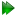Subsections

Pseudo-ellipse equations

Figure 16.15: The pseudo-ellipse model simulated and calculated in-frame Daeg(1) frame order matrix elements. In these plots, θX corresponds to the cone opening half-angle θx, θY to the cone opening half-angle θy and θZ to torsion half-angle σmax. When the half-angle is not varied, the angle is fixed to either θx = π/4, θy = 3π/8 or σmax = π/6. Frame order matrix values have been calculated every 10 degrees. The first angle for the calculated θX and θY graphs is set to 0.01 degrees as a pseudo-ellipse cone opening angle of 0.0 cannot be correctly handled by the numerical integration.Figure 16.16: The pseudo-ellipse model simulated and calculated in-frame Daeg(2) frame order matrix elements. In these plots, θX corresponds to the cone opening half-angle θx, θY to the cone opening half-angle θy and θZ to torsion half-angle σmax. When the half-angle is not varied, the angle is fixed to either θx = π/4, θy = 3π/8 or σmax = π/6. Frame order matrix values have been calculated every 10 degrees. The first angle for the calculated θX and θY graphs is set to 0.01 degrees as a pseudo-ellipse cone opening angle of 0.0 cannot be correctly handled by the numerical integration.Figure 16.17: The pseudo-ellipse model simulated and calculated out-of-frame Daeg(1) frame order matrix elements. In these plots, θX corresponds to the cone opening half-angle θx, θY to the cone opening half-angle θy and θZ to torsion half-angle σmax. When the half-angle is not varied, the angle is fixed to either θx = π/4, θy = 3π/8 or σmax = π/6. Frame order matrix values have been calculated every 10 degrees. The first angle for the calculated θX and θY graphs is set to 0.01 degrees as a pseudo-ellipse cone opening angle of 0.0 cannot be correctly handled by the numerical integration.Figure 16.18: The pseudo-ellipse model simulated and calculated out-of-frame Daeg(2) frame order matrix elements. In these plots, θX corresponds to the cone opening half-angle θx, θY to the cone opening half-angle θy and θZ to torsion half-angle σmax. When the half-angle is not varied, the angle is fixed to either θx = π/4, θy = 3π/8 or σmax = π/6. Frame order matrix values have been calculated every 10 degrees. The first angle for the calculated θX and θY graphs is set to 0.01 degrees as a pseudo-ellipse cone opening angle of 0.0 cannot be correctly handled by the numerical integration.Pseudo-ellipse rotation matrices

For the pseudo-ellipse model, the full torsion-tilt system is used. The full rotation matrix is given in equation 12.74c on page.

Pseudo-ellipse frame order matrix

The frame order matrix isThe surface normalisation factor isPseudo-ellipse 1 degree frame order

The 1 degree frame order matrix with tensor rank-2 consists of the following elementsAs the trigonometric functions of θmax cannot be integrated, these components must be numerically integrated.

Pseudo-ellipse 2 degree frame order

The 2 degree frame order matrix with tensor rank-4 consists of the following elements, using Kronecker product double indices from 0 to 8As the trigonometric functions of θmax cannot be integrated, these components must be numerically integrated. All other frame order matrix elements can be numerically shown to be zero.

Pseudo-ellipse frame order matrix simulation and calculation

The frame order matrix element simulation script from Section 16.2, pagewas used to compare the implementation of equations 16.52 and 16.53 above. Frame order matrix Daeg(1) and Daeg(2) values were both simulated and calculated, both within and out of the motional eigenframe. The in-frame Daeg(1) values are shown in figure 16.15 and Daeg(2) in figure 16.16. The out-of-frame Daeg(1) values are shown in figure 16.17 and Daeg(2) in figure 16.18.

The relax user manual (PDF), created 2020-08-26.